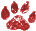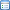# Weekdays

 Namespace: WIN_COM_API
Every once in a while someone needs a way to compute the number of weekdays between two dates. This function evolved from a discussion on the www.universalthread.com.

A function should do one thing and do it well. Therefore this function does not compute holidays. Subtract the output of a custom holidays function from the output of this function to compute the number of business days - which could also be a new function.A function should also be the simplest, cleanest and fastest possible. Ben Creighton came up with a really good one. So I applied formatting and adopted it. ;) -- Mike Yearwood

```*
*  WEEKDAYS.PRG
*  Return the number of days that are MONDAY - FRIDAY
*  in the passed date range WITHOUT LOOPING!
*
*  Author:  Ben Creighton with input from Mike Yearwood and various
*                UniversalThread and Foxite members.
*
*  USAGE:
*    ?WEEKDAYS({^2004-05-01},{^2004-05-31})
*
*  OR:
*    ldFrom = {^2004-05-01}
*    ldTo = {^2004-05-31}
*    lnWeekdays = WEEKDAYS(m.ldFrom, m.ldTo)
*    ?"The number of weekdays was: " + ALLTRIM(STR(m.lnWeekdays)) + "."
*
*  RETURNS:
*    A numeric value for the number of weekdays.
*    This also indicates the routine succeeded.
*    It crashes when called with incorrect parameters.
*
*  lParameters
*     tdDate1 (R)     Start of the date range.
*     tdDate2 (R)     End of the date range.
*
LPARAMETERS m.tdDate1, m.tdDate2
LOCAL lnJ1, lnJ2

lnJ1 = VAL(SYS(11, m.tdDate1))
lnJ2 = VAL(SYS(11, m.tdDate2))

RETURN (INT(m.lnJ2 / 7) - INT(m.lnJ1 / 7)) * 5 ;
- MIN(m.lnJ1 % 7 + 1, 5) ;
+ MIN(m.lnJ2 % 7 + 1, 5)
```

```*The following is my original and now outdated weekeday function - do not use!!!

*
*  WEEKDAYS.PRG
*  Return the number of days that are MONDAY - FRIDAY
*  in the passed date range WITHOUT LOOPING!
*
*  Author:  Mike Yearwood with input from various
*                UniversalThread and Foxite members.
*
*  USAGE:
*    ?WEEKDAYS({^2004-05-01},{^2004-05-31})
*
*  OR:
*    ldFrom = {^2004-05-01}
*    ldTo = {^2004-05-31}
*    lnWeekdays = WEEKDAYS(m.ldFrom, m.ldTo)
*    ?"The number of weekdays was: " + ALLTRIM(STR(m.lnWeekdays)) + "."
*
*  RETURNS:
*    A numeric value for the number of weekdays.
*    This also indicates the routine succeeded.
*    It crashes when called with incorrect parameters.
*
*  lParameters
*     tdFrom (R)     Start of the date range.
*     tdTo   (R)     End of the date range.
*
LPARAMETERS m.tuFrom, m.tuTo

LOCAL ;
ldFrom, ldTo, lnDays, lnDOW

*Martina Jindrova suggested converting DateTimes to Dates.

*I use locals rather than the m.tuFrom and m.tuTo
*because if they were passed by reference, this function
*has no business altering the passed values.

*Part 1 of the formula subtracts Saturday and Sunday from
*the end of the week(s).

**** m.lnDays - (INT(m.lnDays / 7) * 2)

*The rest of the formula drops any leading
*Saturday or Sunday from any partial week.

*We could use IIF() but that just makes
*the formula harder to read/write
*because (m.lnDays % 7) is repeated as is
*SIGN(). Both are calculated every time.
*SIGN() returns 1/0 instead of T/F.

*It seems the formula can be shortened
*without much impact on performance.

*There are three parts to consider.

*Part1 determines if there is a
*partial week.
**** SIGN( (m.lnDays % 7)

*Part2 is looking to drop a leading
*Saturday from that partial week.
**** + m.lnDOW - 7

*Part3 is looking to drop a leading
*Sunday from that partial week.
**** SIGN(m.lnDOW % 7)

*Here's the result table where there are no
*remaining days. Part 1 is 0.
*Day  Part1    Part2   Part3         1+2  3   1+2+3
*Mon -(sign(0 + -6) + sign(1%7)) = -(-1 + 1) =  0
*Tue -(sign(0 + -5) + sign(2%7)) = -(-1 + 1) =  0
*Sat -(sign(0 + -1) + sign(6%7)) = -(-1 + 1) = -0
*Sun -(sign(0 +  0) + sign(7%7)) = -( 0 + 0) = -0

*If there are remaining days, Part 1 is 1.
*Part2 and Part3 trigger on Saturday or Sunday.
*Day  Part1    Part2   Part3         1+2  3   1+2+3
*Mon -(sign(1 + -6) + sign(1%7)) = -(-1 + 1) =  0
*Tue -(sign(1 + -5) + sign(2%7)) = -(-1 + 1) =  0
*Sat -(sign(1 + -1) + sign(6%7)) = -( 0 + 1) = -1 && drop Sat
*Sun -(sign(1 +  0) + sign(7%7)) = -( 1 + 0) = -1 && drop Sun

*Original deprecated formula.
*!*	lnReturn = m.lnDays - (INT(m.lnDays / 7) * 2) ;
*!*		- IIF( m.lnDOW = 7, ;
*!*		     SIGN(m.lnDays % 7), ;
*!*		     SIGN(m.lnDOW - 7 + (m.lnDays % 7)) + 1)

ldFrom = IIF(VARTYPE(m.tuFrom)="T",TTOD(m.tuFrom),m.tuFrom)
ldTo = IIF(VARTYPE(m.tuTo)="T",TTOD(m.tuTo),m.tuTo)
lnDays = (m.ldTo - m.ldFrom) + 1
lnDOW = DOW(m.ldFrom,2)
RETURN m.lnDays - (INT(m.lnDays / 7) * 2) ;
- (SIGN( (m.lnDays % 7) + m.lnDOW - 7) + SIGN(m.lnDOW % 7))```

Contributor: Mike Yearwood, Martina Jindrova
Category UDFA Shorter Function
Try this one using the Julian Day Number function to accomplish the same thing from date1 to date2. The Julian date parameter doesn't require a conversation to date type so datetime passed to date1/date2 works too. If you pass a later date to date1 than date2, the result will be correct AND negative.
```* Calculates weekdays from date1 to date2.
* Includes date1 in the number of weekdays calculation if include_date1=.t.
* If you don't need to have a negative result for date1 > date2 then you can use the 'ALTERNATIVE' lines.

Lparameters date1, date2, include_date1
Local jn1, jn2

jn1 = Val(Sys(11, m.date1))  && ALTERNATIVE:  jn1=Val(Sys(11, Min(m.date1, m.date2)) - iif(include_date1, 1, 0)
jn2 = Val(Sys(11, m.date2))  && ALTERNATIVE:  jn2=Val(Sys(11, Max(m.date1, m.date2))
jn1 = iif(include_date1 AND m.jn1<=m.jn2, m.jn1 - 1, m.jn1)  && ALTERNATIVE:  remove this line
jn2 = iif(include_date1 AND m.jn1>m.jn2, m.jn2 - 1, m.jn2)   && ALTERNATIVE:  remove this line

Return (int(m.jn2 / 7) - int(m.jn1 / 7)) * 5 - Min(m.jn1 % 7 + 1, 5) + Min(m.jn2 % 7 + 1, 5)```

Ben Creighton
Hey Ben

That's exactly what I was looking for when I started the discussion. There had to be a faster way than looping. If you'd be so kind as to modify it to include the start date that would be perfect.

I think this: (INT((m.jn2-m.jn1)/7)+1) instead of: (int(m.jn2 / 7) - int(m.jn1 / 7)) would be good as it is one less division operation. What do you think?

Mike YearwoodUpdate
I added the include date 1 capability above with 'include_date1' parameter. With Regards to reducing the int() part of the equation as suggested, the calculation implicitly depends upon the fact that a Gregorian Calendar Monday is always a multiple of 7 with Julian Day Numbers. This also means on Mondays the remainder is zero when dividing the Julian Day Number by 7. This property makes the shift of the day-of-week of the first week including date1 and the last week including date2 a simpler formula using Min().

Ben CreightonOK. Can't change it that way. How about you handle the alternatives with a compiler directive? Editing the code to change a behavior wouldn't be my first choice. :)

Mike YearwoodYou don't have to use the "alternative" to include the first date in the number of weekdays. That is accomplished by providing a parameter "include_date1" as true or false. The Alternative is strictly if you want to always have the function start on the earlier date and end on the later, regardless of which order the dates are passed.

I created the function, as it stands, with the ability to return negative results if date1 is after date2. The reason is that it is consistent with other date functionality such as GOMONTH() which allows negative months to go backward in time. It is also consistent with the addition and subtraction operators for date math which uses negative numbers to represent going backward in time.

If the 'Alternative' is not useful for you, change nothing. I don't recommend the alternative, but I included it because of how the function at the top of this wiki works.

Ben Creighton
What I mean is there's no point to adding the alternatives. Let both return negatives. :) I already removed that from the original function. I would like to deprecate the original function and upgrade to yours. Co-authors in the comments? -- Mike YearwoodAnother algorithm...
```PROCEDURE WeekDayTest
LPARAM luStart,luEnd,llSixDaysWeek

lnHollyDays=0
* for llSixDaysWeek=.T.
* is hollydays count between luStart and luEnd, exception hollyday in Sunday

* for llSixDaysWeek=.F.
* is hollydays count between luStart and luEnd, exception hollyday in Saturday and Sunday

m.lnDays = ABS(IIF(VARTYPE(m.luStart)="D",m.luStart,TTOD(m.luStart)) -;
IIF(VARTYPE(m.luEnd)="D",m.luEnd,TTOD(m.luEnd))) + 1

m.lnDOWS = DOW(MIN(m.luStart,m.luEnd),2) && Dow of first date
m.lnDOWE = DOW(MAX(m.luStart,m.luEnd),2) && Dow of end date

liFD=IIF(m.llSixDaysWeek,7,6) && first non weekday
lcWD=IIF(m.llSixDaysWeek,",7,",",6,7,") && list of non weekday

IF m.lnDays<=m.liFD-m.lnDOWS
liBDays=IIF(ATC(","+STR(m.lnDOWS,1)+",",m.lcWD)>0,;
0,MIN(m.liFD-m.lnDOWS,m.lnDays)) && before days
lnWDays=MAX(m.liBDays-m.lnHollyDays,0)
ELSE
liBDays=IIF(ATC(","+STR(m.lnDOWS,1)+",",m.lcWD)>0,0,m.liFD-m.lnDOWS) && before days
liADays=IIF(ATC(","+STR(m.lnDOWE,1)+",",m.lcWD)>0,0,m.lnDOWE) && after days
liWeeks=INT((m.lnDays-(m.liBDays+m.liADays))/7) && week count
ENDIF
*?luStart,luEnd,lnWDays
RETURN m.lnWDays
```

-- Martina Jindrova

While this would serve for a specific business days function, IMHO it is better to create and use a "tally" table for such purposes. The table is created for once and all. Then it could be used to find the weekdays, weekends, mondays, tuesdays ... etc in a given range or even complex ranges.

```* Create datesTally table.
* This is done once and could even be embedded into exe
ldStart = date(1900,1,1) && this range is more than needed for many applications.
ldEnd = date(2100,1,1)   && could well be the whole range that VFP recognizes as date.
create Table datesTally free (date d)
FOR ix=0 TO m.ldEnd - m.ldStart
INSERT INTO datesTally VALUES ( m.ldStart + m.ix )
EndFor
Index on date tag date
```

Now that we have tally table that we can use for this and many other purposes here is sample query to return only the weekdays count:

```ldFrom = date(2000,1,1)
ldTo = date()

select count(*) from datesTally ;
where datesTally.date between m.ldFrom and m.ldTo ;
and dow(datesTally,2) between 1 and 5
```

PS: Note that tally table is not created for only counting weekdays. Any program with some scheduling would also need it for many purposes.

Cetin Basoz
( Topic last updated: 2012.07.02 12:00:22 PM )